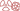THE PHENOMENON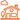IN DAILY LIFE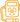QUESTION OF DOSES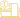AT THE DOCTOR’S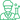At the laboratory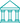At the museum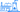NUCLEAR ENERGY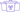A general public knowledge base dedicated to radioactivity created and maintained by the french physics community

# Equivalent Dose

## A local biological dose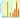Calculating the equivalent dose from the absorbed dose (in grays) takes into consideration the toxicity of the radiations involved for the amount of deposited energy. There is a strog hierarchy among the different types of radiations, with alpha particles proving to be more dangerous when ingested or inhaled than sources of beta or gamma rays. The danger posed by neutrons, which depends on how energetic they are, are still higher but exposures to neutrons are very rare. Protons are only used in rare therapies.

The concept of the “equivalent dose” which takes into account the nature of radiations, is a refinement of the absorbed dose, the local dose introduced first in the history of radioprotection and nuclear medicine to estimate the energy deposited in the human body by radioactive or X-ray sources. The original unit introduced in 1920, was the ‘rad’ which has since been replaced by the gray, a unit worth 100 rads. One gray (Gy) represents 1 joule absorbed per kilogram of matter

In radiotherapies and nuclear therapies, it is the number of grays or milligrays absorbed by an organ or tissues that matters. In the field of radioprotection, however, the concept of absorbed dose is not adequate. For instance, it was observed in the 1950s that a ‘gray‘ of neutrons was ten times more carcinogenic than a ‘gray’ of photons. The absorbed dose of neutrons had to be multiplied by 10 to obtain a new, more helpful quantity, the ‘equivalent dose’.

The equivalent dose is obtained by multiplying the absorbed dose for an organ or body part with a weighting factor – a number that takes into account the nature of the radiations (such as whether they are made up of photons, electrons, neutrons, alpha particles etc) and the severity of the biological damages it causes. The initial equivalent dose unit, the rem (corresponding to the rad), has been replaced by the sievert, which is equivalent to 100 rems.

The weighting factors have empirical values which reflect the current knowledge in radioprotection . The less damaging particles are the less ionizing ones, photons and electrons.

In the field of nuclear diagnostics, where beta and gamma rays are used, the equivalent doses are therefore numerically identical to the absorbed doses, though the two have different units. In these situations, however, it has become more common to talk about the value in sieverts.

Another source of confusion is that the sievert serves as the unit for two different quantities: the equivalent dose, used to determine the dose absorbed by a specific organ or body part, and the effective dose, used to determine the effect on the whole body.THE PHENOMENONIN DAILY LIFEQUESTION OF DOSESAT THE DOCTOR’SAt the laboratoryAt the museumNUCLEAR ENERGY